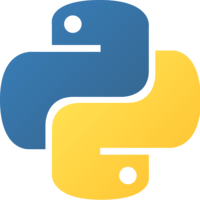1×
Become a member
to unlock all featuresLevel Up!

Access all courses & lessons on egghead today and lock-in your price for life.

Autoplay

Use Linear Regression To Estimate Continuous Values with Python and Scikit-learnScikit-Learnpython

Linear regression is a linear model that is used for regression problems, or problems where the goal is to predict a value on a continuous spectrum (as opposed to a discrete category).

We’ll use linear regression to estimate continuous values. In this case, we’ll predict house prices in Boston. We'll also look at how to visualize our results with matplotlib, and how to evaluate our models with different metrics for regression problems.CodeYou must be a Member to view code

Access all courses and lessons, track your progress, gain confidence and expertise.

Become a Member
and unlock code for this lessonDiscussTranscript

Instructor: We're going to import mapplotlib.pyplot as plt for visualization later. From scikit-learn, which is sklearn, we'll import scikit-learn's datasets. We'll import metrics to evaluate our model. From sklearn.model_selection, we'll import train-test split, which will allow us to make training and test data. Finally, from sklearn.linear_model, we'll import linearRegression.

The first thing we want to do is load in our dataset, and scikit-learn has in-built datasets that we'll be using. First, we'll be working with Boston housing prices. To load this dataset, we say datasets.load_boston. It's always a good idea to explore the dataset a little bit, let's see what this dataset contains.

We can print boston.keys, and my filename here is linear.py. We can see four keys here -- data, which is the data, our feature_names, which are the feature names, descr, which is the describing the data set, and target, which are our target labels.

Let's example these a little bit more. We can say print boston.feature_names, and we can see 13 variables here. If we print boston.description, we can take a look at some of these features. We can see that the features are things like crime rate, whether it's next to the river, the average number of rooms, the distance to work, et cetera.

Let's also look at the data. We'll just look at the first five data points. Let's also look at the target values. We can see that each data point has 13 variables and that the target is one number, which is the housing price, in the thousands. This is old data. We're using these 13 variables to predict the housing price in the thousands.

One more thing we can do is we can print boston.data.shape and the boston.target.shape. We can see that there are 506 data points, with 13 variables each. For the target, there are 506 single data points. Great.

We're going to assign x to be boston.data, and y will be boston.target, our target labels. One important component of machine learning is to have training data and test data. We can create this with the train-test split function. We'll say x_train, x_test, y_train, and y_test equals train-test split.

This takes x, y, the proportion of data that we want to go into our test data, and this number can vary, though you want your training data to be significantly larger. We'll say a third of the data can go into testing. The last thing is to add this optional randomState argument. This will just make it deterministic so that the data will get split the same way every time we run it. This can be literally any number, we'll just say 16.

From there, we just say model equals LinearRegression, and model.fit, x_train, y_train. That's all there is to it. Then, if we want to use this model to make predictions, we say model.predict our test data. We can see a whole bunch of housing predictions here.

We can visualize these by saying plt.scatter, our actual y test data labels versus the predictive labels. We'll add an x label, which is the actual prices and a y label, which is the predicted prices. Then, we say plt.show. We can see here that this looks pretty good. It's not perfectly linear, but the model's predicting the prices OK.

From here, we'll look at a couple of metrics that will help us evaluate this model. Most models have an in-built score, which you can access by typing model.score and passing in the x test data and the y test labels.

For linear regression, the in-built score is based on r^2, also known as the coefficient of determination. This is a number between zero percent and 100 percent that basically describes how well the model fits the data. Generally, though not always, it's better to have a higher r^2 number, and that's worth reading more on.

There's also the mean squared error, which can be found by typing metrics.mean_squared_error and passing the y test data, or the actual labels, and the predicted labels. This is a metric that calculates the error of the model. It's generally better for it to be lower. Here, we can see our r^2 gave us about 64 percent, and our mean squared error is 23.4.